Related Articles

# Mimic the Linux adduser command in C

• Last Updated : 13 Jul, 2021

Programming for fun can manifest itself in the works of leisurely coding practice as well. Regardless of being academically inadequate, the task certainly does contribute to the programmer’s understanding of the resourcefulness of the language. Here let’s look at a distinct programming activity that also translates into a low-level insight and fluency building exercise.

Problem Statement: Mimic the adduser command on Linux in order to create an Ordinary or System User using C Program as the programming language. Here’s what the question implicitly demands as a solution so that the programmer adheres to the formal execution of the command:

• One must provide a precise replication of the CLI on a Linux terminal during execution.
• One must handle a passwd file.
• One must also handle a shadow file.
• The execution of both files must be governed by specifying the PFILE environment variable.

Prerequisites: Let’s have a quick look at the prerequisites before starting to work on the code. First, start by grasping the idea behind the passwd file. Also, feel free to refer to the Linux man-pages.

1. The passwd file: Technically represented as the /etc/passwd file, this file’s prime purpose is to monitor or store a record of registered users who have been granted access to the system. It features a record of 7 important fields.UID is essentially the User ID and GID is the Group ID. Meanwhile, GECOS is the field that is used to store the user’s real name and phone number. The Login Shell is a field responsible for reading files and setting the environment variables.

Each of these fields is separated by a colon. For the purpose of this program, details will be stored until the GECOS field. Nevertheless, readers are encouraged to explore further to incorporate the other fields as well.

2. The shadow file: Formally defined as the /etc/shadow file, this file plays a role similar to the passwd file. However, it focuses on everything related to the encrypted password in the passwd file. Unlike the passwd file, it is only readable by the root user. Typically, it features a record of 9 fields.The Password must remain in its encrypted format – here is an interesting bit where we will be developing our own encrypting algorithm (basic one). The 3rd and 4th fields refer to the minimum and maximum gap in days between password changes (0 by default). The 5th and 6th fields define how long before the password expiration and disabling account notifications must be prompted by the user. All these fields are completely customizable. Remember to encrypt all data entering this file.

3. The PFILE ENV: The purpose of environment variables is to affect the way a program or software would behave otherwise. For example, the \$LANG variable would communicate to software that the user understands a particular language, and therefore it must provide the same language at the interface level. Here we shall use a PATH variable to mimic the location that the system looks for the passwd and shadow files. We shall assign it the name PFILE.

Command Options: Upon typing useradd on the terminal, it is customary to specify another value known as the ‘option’. The options could be various letters. However, to limit this program, use the -r and the -m options only.

• The -r option creates a system user.
• The -m option associates a home directory with the user’s account.

Syntax: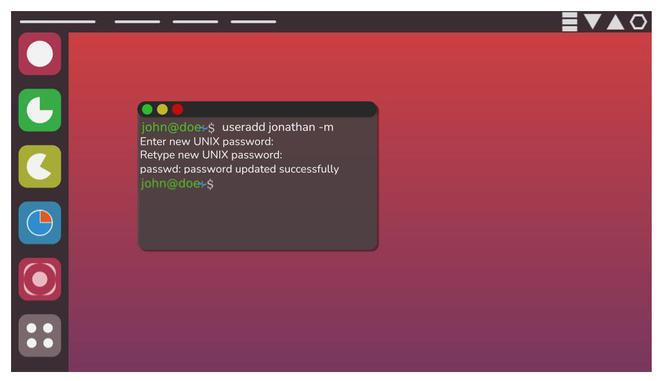Note:

• useradd is 7 characters long.
• useradd is followed by a space and then the username which extends till the next space character is reached.
• Following the second space, the character is a hyphen and the option character.
• Once the command is entered, the password is set.

Below are the steps to implement it using C program:

1. The main.c: Here, specify all the header files and the variables and give all function calls. It shall serve as a blueprint for the compiler.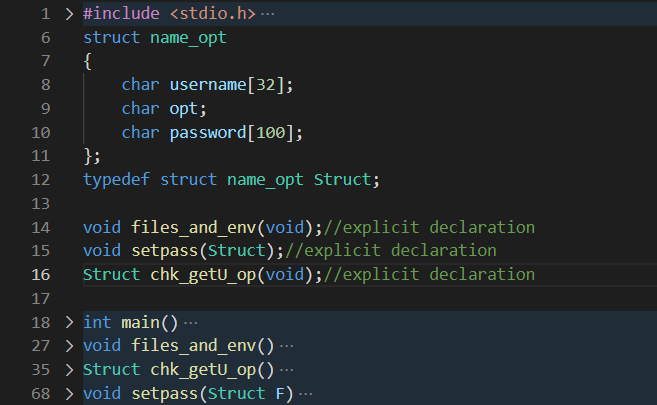A quick look at the layout of the code to follow.

Here, define a struct that shall hold all the data that must be updated. Any other method would introduce additional lines of code and make tracing the code difficult. Also, take it as great advice to always rename the struct you create. When using struct as parameters and return types, not renaming will cause substantial amounts of referencing and identifier errors during compilation.

int main() is the base where all functions are called and the procedure is loaded out. void files_and_env() is where the PATH variable is assigned to PFILE and initializes the files. Remember to insert a valid location or path of the files created for f1 and f2. Moving on, Struct chk_getU_op() is a function that checks the input command on the terminal, gets the username out of the command as well as the option. It returns an object of type struct

Note: Use a capital S in Struct (when declaring return type) since typedef is being used to rename the struct in line 12. Finally, void setpass() prompts the user to associate a password with the new user that has been created. This function is parameterized i.e., it takes in the object that was returned from chk_getU_op() earlier.

Below is the C program to implement the above approach:

## C

 `// C program to implement``// the above approach``#include "server.h"``#include ``#include ``#include ``#include ``struct` `name_opt {``    ``char` `username;``    ``char` `opt;``    ``char` `password;``};`` ` `typedef` `struct` `name_opt Struct;`` ` `// Explicit declaration``void` `files_and_env(``void``);``void` `setpass(Struct);``Struct chk_getU_op(``void``);`` ` `// Driver code``int` `main()``{``    ``Struct fn;`` ` `    ``// Additional character denoting``    ``// the option``    ``char` `opt;`` ` `    ``// Function to declare files and``    ``// set environment variables``    ``files_and_env();``    ``fn = chk_getU_op();``    ``setpass(fn);``    ``return` `(0);``}`` ` `void` `files_and_env()``{``    ``FILE` `*f1, *f2;`` ` `    ``// Assigning environment variable``    ``// PFILE for protecting and``    ``// restricting access``    ``putenv(``        ``"PFILE = -/Environment/rms"``);`` ` `    ``// Displays the path of the``    ``// environment variable``    ``printf``(``"PFILE: %s\n"``,``           ``getenv``(``"PFILE"``));``    ``f1 = ``fopen``(``"Location of file\\passwd.txt"``, ``"r"``);``    ``f2 = ``fopen``(``"Location of file\\shadow.txt"``, ``"r"``);``}`` ` `Struct chk_getU_op()``{``    ``int` `i = 8;``    ``int` `ctr = 0;``    ``int` `x, l = 0;``    ``char` `command[] = { ``'u'``, ``'s'``, ``'e'``, ``'r'``,``                       ``'a'``, ``'d'``, ``'d'` `};`` ` `    ``// Linux accepts only 42 char``    ``// usernames``    ``char` `FunctionCall;``    ``Struct send;``    ``gets``(FunctionCall);`` ` `    ``for` `(x = 0; x < 7; x++) {``        ``if` `(FunctionCall[x] == command[x]) {``            ``ctr += 1;``        ``}``    ``}``    ``if` `(ctr != 7) {``        ``printf``(``"Invalid command.\n"``);``        ``exit``(0);``    ``}``    ``else` `{``        ``// Ends when space char is reached``        ``while` `((``int``)FunctionCall[i] != 32) {``            ``send.username[i - 8] = FunctionCall[i];``            ``i++;``        ``}``        ``l = ``strlen``(send.username);``        ``send.opt = FunctionCall[10 + l];``    ``}``    ``return` `send;``}`` ` `void` `setpass(Struct F)``{``    ``char` `pass;``    ``char` `UID;``    ``printf``(``"Changing password for user.....\n"``);``    ``printf``(``"New UNIX password: "``);``    ``scanf``(``"%s\n"``, *(F.password));`` ` `    ``if` `(F.opt == ``'r'``) {``        ``printf``(``"UID: "``);``        ``scanf``(``"%s"``, UID);``        ``if` `(``strlen``(UID) == 2) {``            ``// Calling password function``            ``passwd(F.username, F.password,``                   ``UID);`` ` `            ``// Calling shadow function``            ``shadow(F.username, F.password,``                   ``UID);``        ``}`` ` `        ``// When the UID is not 2 characters long``        ``else` `{``            ``while` `(``strlen``(UID) != 2) {``                ``printf``(``"Invalid UID. Retype UID: "``);``                ``scanf``(``"%s"``, UID);``            ``}`` ` `            ``// Calling password function``            ``passwd(F.username, F.password,``                   ``UID);`` ` `            ``// Calling shadow function``            ``shadow(F.username, F.password,``                   ``UID);``        ``}``    ``}`` ` `    ``// If the option is 'm'``    ``else` `{``        ``printf``(``"UID: "``);``        ``scanf``(``"%s"``, UID);``        ``if` `(``strlen``(UID) <= 2) {``            ``printf``(``"Normal Users not allowed this UID!\n"``);``            ``printf``(``"Retype UID: "``);``            ``scanf``(``"%s"``, UID);``            ``passwd(F.username, F.password, UID);``            ``shadow(F.username, F.password, UID);``        ``}``        ``else` `{``            ``passwd(F.username, F.password, UID);``            ``shadow(F.username, F.password, UID);``        ``}`` ` `        ``// end of setpass``    ``}`` ` `    ``// end of main.c``}`

2. The passwd.c file: Here are the details of the username are stored that has just been created. The data is written on the passwd.txt file. Use another array declared in the function to mask the contents of the password that was input in the setpass() function earlier.

Below is the C program to implement the above approach:

## C

 `// C program to implement``// the above approach``#include "server.h"``#include ``#include ``#include `` ` `void` `passwd(``char``* username,``            ``char``* password,``            ``char``* UID)``{``    ``int` `len, k = 0;`` ` `    ``// Pointing to the password file``    ``// that will now be appended``    ``FILE``* fs;``    ``fs = ``fopen``(``"Location of the file\\passwd.txt"``,``               ``"+a"``);``    ``len = ``strlen``(password);``    ``char` `temp_passwrd[len];`` ` `    ``for` `(k = 0; k < len; k++) {``        ``// Since the passwd may not``        ``// reveal user-sensitive info``        ``temp_passwrd[k] = ``'\$'``;``    ``}`` ` `    ``fprintf``(fs, ``"%s:%c:%s:%s\n"``, username,``            ``'x'``, temp_passwrd, UID);``    ``fclose``(fs);`` ` `    ``// end of passwd.c``}`

The passwd.txt file must look somewhat like this after execution: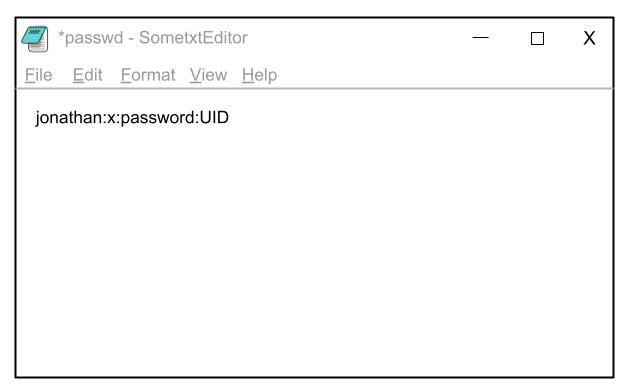3. The shadow.c file: As mentioned earlier, this file’s main function is to encrypt the password as it is received as input from the terminal. Readers must experiment with different encrypting techniques rather than simple alphanumeric schemes. However, developing a very basic technique such as the one below is a good start.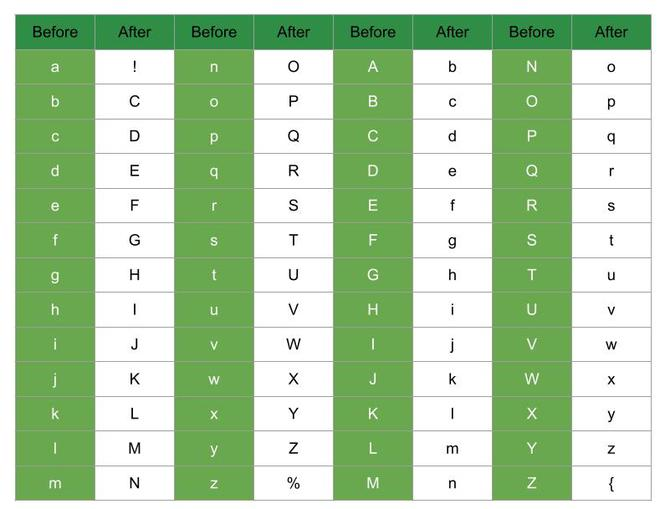Below is the C program to implement the above approach:

## C

 `// C program to implement``// the above approach``#include "server.h"``#include ``#include ``#include ``#include ``#include ``#include `` ` `void` `shadow(``char``* username,``            ``char``* password,``            ``char``* UID)``{``    ``char` `epass;`` ` `    ``// File pointer``    ``FILE``* fp1;`` ` `    ``fp1 = ``fopen``(``"Location of file\\shadow.txt"``,``                ``"+a"``);`` ` `    ``// Call the encryption function``    ``Encryption(epass, fp1, username, UID);``}`` ` `void` `Encryption(``char``* epass, ``FILE``* fp1,``                ``char``* username, ``char``* UID)``{``    ``// Integer that will help in indexing``    ``int` `x = 0;``    ``char``* e = epass;``    ``char` `encrypt;`` ` `    ``// While is prefered over for loops here``    ``while` `(*e) {``        ``if` `(``islower``(*e) == ``true``) {``            ``if` `(*e == ``'a'``) {``                ``// a becomes !``                ``encrypt[x] = ``'!'``;``            ``}``            ``else` `if` `(*e == ``'z'``) {``                ``// z becomes %``                ``encrypt[x] = ``'%'``;``            ``}``            ``else` `{``                ``// Make capital``                ``encrypt[x] = ``toupper``((``char``)((``int``)*e) + 1);``            ``}``        ``}`` ` `        ``// If we have upper case letters``        ``else` `{``            ``// Increment and make lower``            ``encrypt[x] = ``tolower``((``char``)(((``int``)*e) + 1));``        ``}``        ``e++;``        ``x++;`` ` `        ``// end of while``    ``}`` ` `    ``// end of shadow.c``}`

The change in the shadow.txt file will be apparent as shown: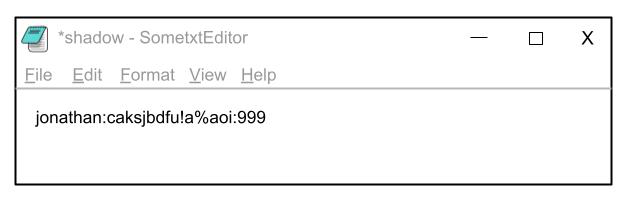Notice the server.h header in all three source code files. This is a file referencing all the functions in the passwd.c and the shadow.c file. The reason behind including such a file is that the main.c file stays informed of where the passwd, shadow, and Encryption files are located. This is what the server.h file contains: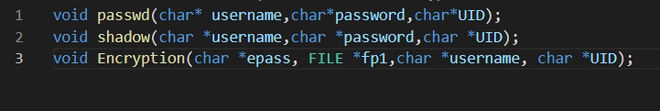A good practice to ensure sequential execution and appropriate compilation of multiple source code files is to use makefiles. Makefiles help with keeping all source code files compiled at the point of execution so that upon editing any other source code, other files remain ready for execution. Readers are encouraged to develop this habit.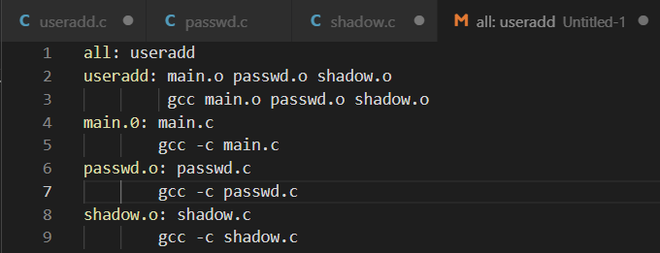Want to learn from the best curated videos and practice problems, check out the C Foundation Course for Basic to Advanced C.

My Personal Notes arrow_drop_up